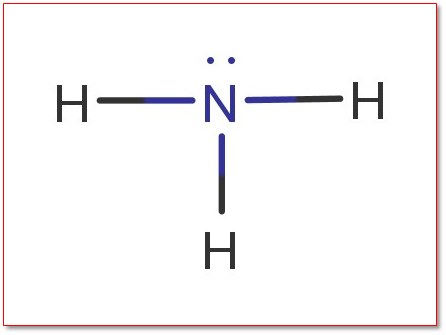# Problem: Why is the H–N–H angle in NH3 smaller than the H–C–H bond angle in CH4? Why is the H–N–H angle in NH4+ identical to the H–C–H bond angle in CH4?

###### FREE Expert Solution

Electron Regions          Electronic Geometry          Bond Angles

2                                      linear                                    180˚

3                                      trigonal planar                      120˚

4                                      tetrahedral                            109.5˚

5                                      trigonal bipyramidal              90˚, 120˚, and 180˚

6                                      octahedral                            90˚ and 180˚

For NH3N is the central atom

Total valence electrons:

Group             Valence Electrons

N           5A                   1 × 5 e5 e

H           1A                   3 × 1 e3 e

Total:  8 valence e

Lewis Structure:96% (231 ratings)###### Problem Details

Why is the H–N–H angle in NH3 smaller than the H–C–H bond angle in CH4? Why is the H–N–H angle in NH4identical to the H–C–H bond angle in CH4?